# How Things Float

How Things Work series

Last Updated: September 2020Overview

Why do things float or sink? Objects float when they are positively buoyant, or less dense than the fluid in which they are immersed. Archimedes Principle can explain this phenomenon – “When an object is immersed fully or partially in a fluid, it experiences an upward force that is equal to the weight of the fluid displaced by it.” Objects need to have a greater ratio of empty space to mass than the fluid. Basic understanding of buoyancy, density, fluids, and the Archimedes Principle are necessary in order to understand how things float.Buoyancy

Buoyancy is a force that moves an object upward. This upward force occurs when the object is immersed (either fully or partially) in a fluid that has measurable density. The fluid can be a liquid or a gas (not a solid). Buoyant force is measured in Newtons (N) in the International System of Units (SI).Types of Buoyancy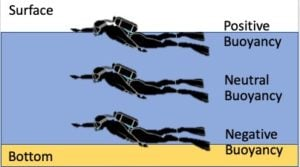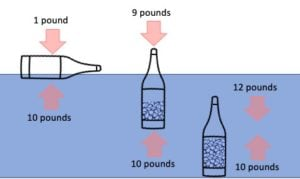1. NEGATIVE BUOYANCY –  An object is negatively buoyant when it is denser than the fluid it displaces. The object will sink because its weight is greater than the buoyant force.
2. NEUTRAL BUOYANCY  - An object is neutrally buoyant when its density is equal to the density of the fluid in which it is immersed, resulting in the buoyant force balancing the force of gravity that would otherwise cause the object to sink or rise. An object that has neutral buoyancy will neither sink nor rise.
3. POSITIVE BUOYANCY – An object is positively buoyant when it is lighter than the fluid it displaces. The object will float because the buoyant force is greater than the object’s weight.Density

Density is a measure of how much matter occupies a given amount of space. High density substances have tightly packed particles and low density substances are made up of loosely packed particles.The density, of a substance is its mass per unit volume. The symbol most often used for density is ρ, the Greek symbol Rho. Mathematically, density is defined as mass divided by volume: where ρ is the density, m is the mass, and v is the volume.

ρ = m / v

Compare a sponge and a rock of the same size. The rock is more dense because the sponge has hundreds of holes in the same volume of space. In the case of the sponge, the mass of the sponge as well as the air that fills the holes of the sponge is measured. Using this same principal, a boat hull full of air will float, but a boat hull full of water will sink. The density of the air-filled boat is much less than a boat hull filled with water.Fluids

Fluids are a state of matter, such as liquid or gas, in which the component particles (generally molecules) can move past one another. Fluids flow easily and conform to the shape of their containers. It is common to describe liquids (water, juice, coolant) as fluids, and gases (such air, nitrogen, propane) as a gas. However, liquids and gases share common properties, such as compressibility, density, pressure, buoyancy and viscosity, and they are scientifically classified together as fluids.Archimedes Principle

Archimedes’ principle states that the upward buoyant force that is exerted on a body immersed in a fluid, whether fully or partially submerged, is equal to the weight of the fluid that the body displaces.

Mass out of fluid – Mass in fluid = Density of fluid * Volume of solid

Buoyancy (N) = ρ * v

1st part: When an object is immersed fully or partially in a fluid, it experiences an upward force that can be seen and felt.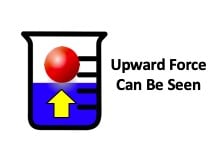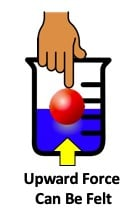2nd part: The upward force is equal to the weight of the fluid displaced by the object.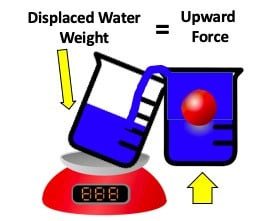Archimedes, a famous Greek scientist, was given the task of determining whether the king’s golden wreath, a gift to the gods, was made of real gold or some alloy. Archimedes wanted to melt the gold material into a known volume and weigh it, thus determining the density. But the king would not allow this. Archimedes thought about this for some time, until one day while bathing, he noticed the water level increased as he entered the tub, proportionally to his volume. This meant that he could put the difficult-to-measure crown in water and instantly know the volume of the crown by measuring the displacement of water. At this point, Archimedes ran through the streets shouting “Eureka! Eureka!”

Archimedes determined that by measuring the weight of the water increase when the wreath was submerged, he was actually measuring the buoyant force acting on the wreath. And from this, he could divide by the density of water, and find the volume of the crown. Fun Fact: Buoy = float (in Latin).Vocabulary

Archimedes Principle: The relationship between buoyancy and displaced fluid: An immersed object is buoyed up by a force equal to the weight of the fluid it displaces.

Buoyancy:  An upward force acting on an immersed or floating object by the supporting fluid.

Equilibrium:  A state of balance between opposing forces.

Force:   A push or a pull.  An influence on a body or system, causing or tending to cause a change in movement or shape.

Mass:  The amount of matter that is contained by an object.

Negative Buoyancy:  Exists when the weight of the body is greater than the weight of an equal volume of the displaced fluid. The body sinks.

Neutral Buoyancy:   Exists when the weight of the body is equal to the weight of an equal volume of the displaced fluid. The body remains suspended – neither rising nor sinking – unless acted upon by an outside force.

Newton (N): The derived unit used to measure force in the International System of Units (SI). One newton is equal to the force needed to accelerate a mass of one kilogram one meter per second per second.

Positive Buoyancy:   Exists when the weight of the body is less than the weight of an equal volume of the displaced fluid.

Pressure:  The force per unit of surface area; exerted perpendicular to the surface; measured in Pascals.

Volume:  The amount of space enclosed by a shape or object; how much 3-dimensional space (length, width, and height) it occupies.

Weight:  The downward force caused by gravity on an object.1. A dog jumps into a pool. The water holds him up because he only weighs 10N in water. On land, he weighs 45N. What is the buoyant force?
2. A person weighs 250N while swimming in the Dead Sea. When outside of the water, they weigh 600N. What is the buoyant force acting on them? Will they sink or float?
3. A small fish weighing 27 g displaced a volume of water that weighed 25N. the weight of the fish was 30N. What is the buoyant force on the fish and will it sink or float?
4. A rock has a mass of 76 g and displaced 80 mL of water when it was submerged. What is the volume of the rock?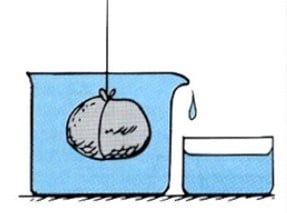Resources

Videos:

How Submarines Work (How Stuff Works): Provides a background on submarines from how they dive and surface to how they maintain hospitable conditions for the people who live and work on the vessel.

Mythbusters: “Let’s Talk Buoyancy” (Discovery Go): A video where the Mythbusters explain buoyancy and relate it to a pirate myth of turning a rowboat into a submarine.

Buoyancy Playground (University of Colorado – Boulder): A buoyancy simulator supported by the University of Colorado Boulder that allows you to manipulate different experimental parameters including mass, volume, and density of the object and the fluid. The simulator provides quantitative measurements and allows you to overlay force vectors onto the simulation.

Underwater Exploration (National Geographic): A National Geographic website that provides information on the many expeditions and discoveries made by National Geographic explorers. Includes articles, videos, and other multimedia sources.

Float or Sink (Learning Junction – YouTube):  A video on the concept of floating or sinking (density) for young children.

Buoyancy: What Makes Something Float or Sink (Kids Want to Know – YouTube):  A short overview video about buoyancy.

Lesson Plans:

Exploring Buoyancy – Design Challenge Learning (The Tech: Museum of Innovation)

Lesson: Estimating Buoyancy (University of Colorado – Teach Engineering STEM Curriculum for K-12)

Lesson: Archimedes’ Principle, Pascal’s Law and Bernoulli’s Principle (University of Colorado – Teach Engineering STEM Curriculum for K-12)## Related Resources#### Electricity

This resource is a part of the How Things Work series.#### Electric Motors

This resource is a part of the How Things Work series.#### Microcontrollers

This resource is a part of the How Things Work series.#### PVC

This resource is a part of the How Things Work series.#### Relays

This resource is a part of the How Things Work series.#### Switches

This resource is a part of the How Things Work series.